Home » Statistics for Data Science: What is Skewness and Why is it Important?

# Statistics for Data Science: What is Skewness and Why is it Important?

## Overview

• Skewness is a key statistics concept you must know in the data science and analytics fields
• Learn what is skewness, and why it’s important for you as a data science professional

## Introduction

The concept of skewness is baked into our way of thinking. When we look at a visualization, our minds intuitively discern the pattern in that chart.

As you might already know, India has more than 50% of its population below the age of 25 and more than 65% below the age of 35. If you’ll plot the distribution of the age of the population of India, you will find that there is a hump on the left side of distribution and the right side is comparatively planar. In other words, we can say that there’s a skew towards the end, right?

So even if you haven’t read up on skewness as a data science or analytics professional, you have definitely interacted with the concept on an informal note. And it’s actually a pretty easy topic in statistics – and yet a lot of folks skim through it in their haste of learning other seemingly complex data science concepts. To me, that’s a mistake.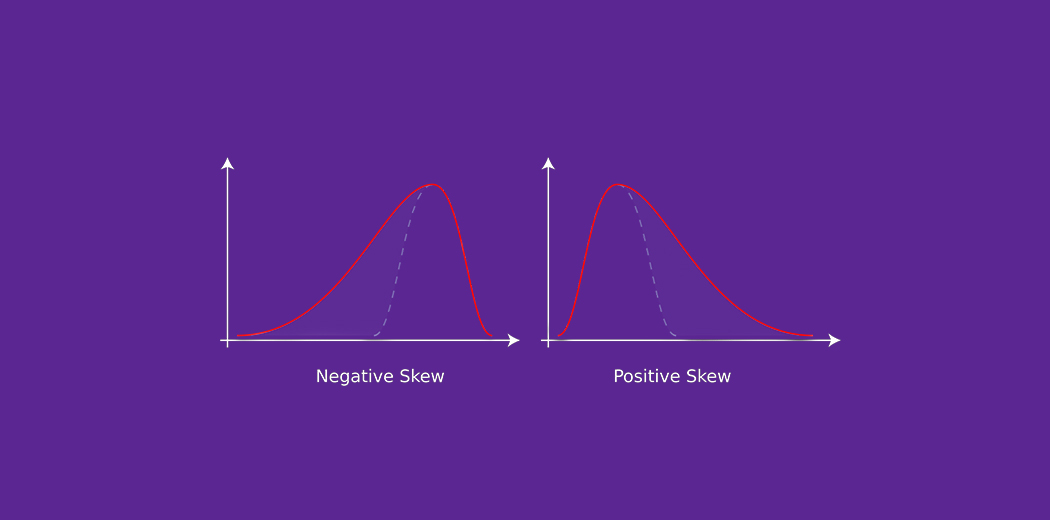Skewness is a fundamental statistics concept that everyone in data science and analytics needs to know. It is something that we simply can’t run away from. And I’m sure you’ll understand this by the end of this article.

Here, we’ll be discussing the concept of skewness in the easiest way possible. You’ll learn about skewness, its types, and its importance in the field of data science. So buckle up because you’ll learn a concept that you’ll value during your entire data science career.

Note: Here are a couple of resources to help you dive deeper into the world of statistics for data science:

• What is Skewness?
• Why is Skewness Important?
• What is a Normal Distribution?
• Understanding Positively Skewed Distribution
• Understanding Negatively Skewed Distribution

## What is Skewness?

Skewness is the measure of the asymmetry of an ideally symmetric probability distribution and is given by the third standardized moment. If that sounds way too complex, don’t worry! Let me break it down for you.

In simple words, skewness is the measure of how much the probability distribution of a random variable deviates from the normal distribution. Now, you might be thinking – why am I talking about normal distribution here?

Well, the normal distribution is the probability distribution without any skewness. You can look at the image below which shows symmetrical distribution that’s basically a normal distribution and you can see that it is symmetrical on both sides of the dashed line. Apart from this, there are two types of skewness:

• Positive Skewness
• Negative Skewness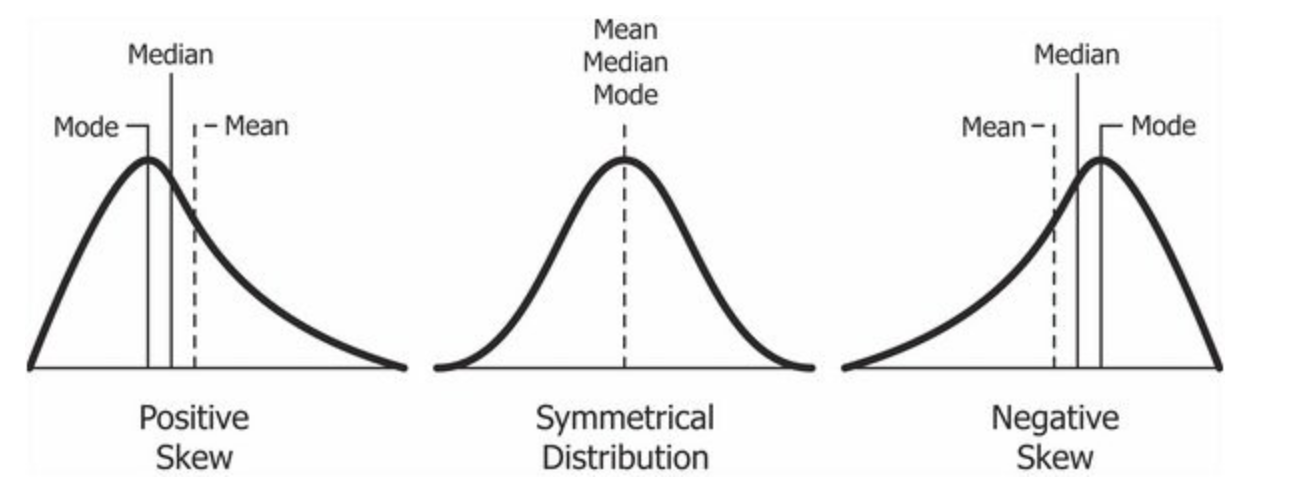Credits: Wikipedia

The probability distribution with its tail on the right side is a positively skewed distribution and the one with its tail on the left side is a negatively skewed distribution. If you’re finding the above figures confusing, that’s alright. We’ll understand this in more detail later.

Before that, let’s understand why skewness is such an important concept for you as a data science professional.

## Why is Skewness Important?

Now, we know that the skewness is the measure of asymmetry and its types are distinguished by the side on which the tail of probability distribution lies. But why is knowing the skewness of the data important?

First, linear models work on the assumption that the distribution of the independent variable and the target variable are similar. Therefore, knowing about the skewness of data helps us in creating better linear models.

Secondly, let’s take a look at the below distribution. It is the distribution of horsepower of cars: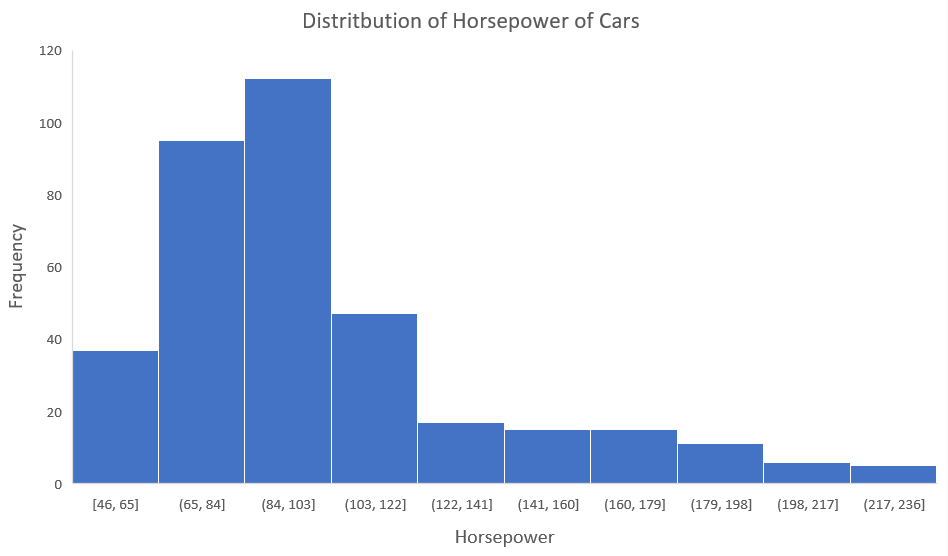You can clearly see that the above distribution is positively skewed. Now, let’s say you want to use this as a feature for the model which will predict the mpg (miles per gallon) of a car.

Since our data is positively skewed here, it means that it has a higher number of data points having low values, i.e., cars with less horsepower. So when we train our model on this data, it will perform better at predicting the mpg of cars with lower horsepower as compared to those with higher horsepower.

Also, skewness tells us about the direction of outliers. You can see that our distribution is positively skewed and most of the outliers are present on the right side of the distribution.

Note: The skewness does not tell us about the number of outliers. It only tells us the direction.

Now we know why skewness is important, let’s understand the distributions which I showed you earlier.

## What is Symmetric/Normal Distribution?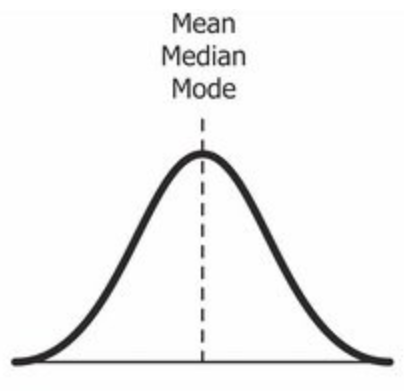Credits: Wikipedia

Yes, we’re back again with the normal distribution. It is used as a reference for determining the skewness of a distribution. As I mentioned earlier, the ideal normal distribution is the probability distribution with almost no skewness. It is nearly perfectly symmetrical. Due to this, the value of skewness for a normal distribution is zero.

But, why is it nearly perfectly symmetrical and not absolutely symmetrical?

That’s because, in reality, no real word data has a perfectly normal distribution. Therefore, even the value of skewness is not exactly zero; it is nearly zero. Although the value of zero is used as a reference for determining the skewness of a distribution.

You can see in the above image that the same line represents the mean, median, and mode. It is because the mean, median, and mode of a perfectly normal distribution are equal.

So far, we’ve understood the skewness of normal distribution using a probability or frequency distribution. Now, let’s understand it in terms of a boxplot because that’s the most common way of looking at a distribution in the data science space.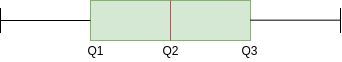The above image is a boxplot of symmetric distribution. You’ll notice here that the distance between Q1 and Q2 and Q2 and Q3 is equal i.e.: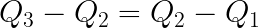But that’s not enough for concluding if a distribution is skewed or not. We also take a look at the length of the whisker; if they are equal, then we can say that the distribution is symmetric, i.e. it is not skewed.

Now that we’ve discussed the skewness in the normal distribution, it’s time to learn about the two types of skewness which we discussed earlier. Let’s start with positive skewness.

## Understanding Positively Skewed Distribution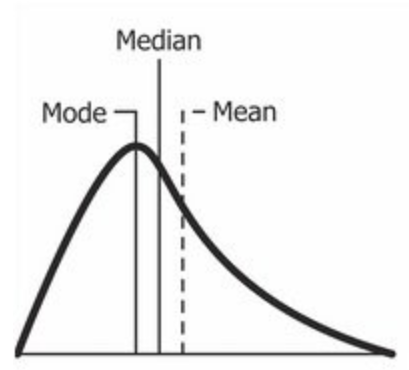Source: Wikipedia

A positively skewed distribution is the distribution with the tail on its right side. The value of skewness for a positively skewed distribution is greater than zero. As you might have already understood by looking at the figure, the value of mean is the greatest one followed by median and then by mode.

So why is this happening?

Well, the answer to that is that the skewness of the distribution is on the right; it causes the mean to be greater than the median and eventually move to the right. Also, the mode occurs at the highest frequency of the distribution which is on the left side of the median. Therefore, mode < median < mean.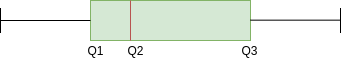In the above boxplot, you can see that Q2 is present nearer to Q1. This represents a positively skewed distribution. In terms of quartiles, it can be given by: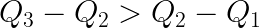In this case, it was very easy to tell if the data is skewed or not. But what if we have something like this: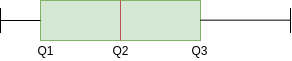Here, Q2-Q1 and Q3-Q2 are equal and yet the distribution is positively skewed. The keen-eyed among you will have noticed the length of the right whisker is greater than the left whisker. From this, we can conclude that the data is positively skewed.

So, the first step is always to check the equality of Q2-Q1 and Q3-Q2. If that is found equal, then we look for the length of whiskers.

## Understanding Negatively Skewed Distribution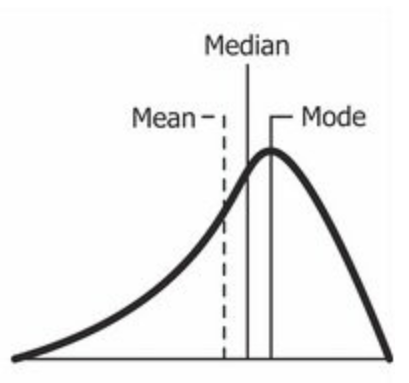Source: Wikipedia

As you might have already guessed, a negatively skewed distribution is the distribution with the tail on its left side. The value of skewness for a negatively skewed distribution is less than zero. You can also see in the above figure that the mean < median < mode.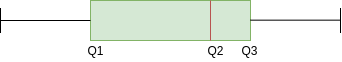In the boxplot, the relationship between quartiles for a negative skewness is given by: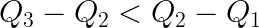Similar to what we did earlier, if Q3-Q2 and Q2-Q1 are equal, then we look for the length of whiskers. And if the length of the left whisker is greater than that of the right whisker, then we can say that the data is negatively skewed.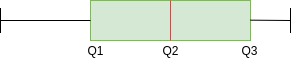## How Do We Transform Skewed Data?

Since you know how much the skewed data can affect our machine learning model’s predicting capabilities, it is better to transform the skewed data to normally distributed data. Here are some of the ways you can transform your skewed data:

• Power Transformation
• Log Transformation
• Exponential Transformation

Note: The selection of transformation depends on the statistical characteristics of the data.

## End Notes

In this article, we covered the concept of skewness, its types and why it is important in the data science field. We discussed skewness at the conceptual level, but if you want to dig deeper, you can explore its mathematical part as the next step.

Also, you can read articles on the other important topics of statistics:

Connect with me in the comments section below if you have any queries.

You can also read this article on our Mobile APP•Sergii says:

Good job!
It’s very simple article for understanding and author has done this on highest mark!

•Ashok says:

Very nice explanation… Thanks for the article

•mugo says:

good work

•S.Debroy says:

Great article….. Fundamental is always important…..

•Martins says:

Nice, well explained